Click to Chat

1800-1023-196

+91-120-4616500

CART 0

• 0

MY CART (5)

Use Coupon: CART20 and get 20% off on all online Study Material

ITEM
DETAILS
MRP
DISCOUNT
FINAL PRICE
Total Price: Rs.

There are no items in this cart.
Continue Shopping• Complete JEE Main/Advanced Course and Test Series
• OFFERED PRICE: Rs. 15,900
• View Details

```Revision Notes on Modern Physics

Atomic Physics

e/m of an electron (Thomson Method):-

(a) e/m of a particle is called the specific charge of the particle.

e/m = v/rB

Here, r is the radius of curvature, B is the strength of magnetic field, v is the velocity, e is the charge on cathode ray particle and m is  the mass.

(b) v = E/B

Electric field:- E = V/d

Photo electric effect:- Photo-electric effect is the phenomenon of emission of electrons from the surfaces of certain substances, mainly metals, when light of shorter wavelength is incident upon them.

Effect of collector’s potential on photoelectric current:-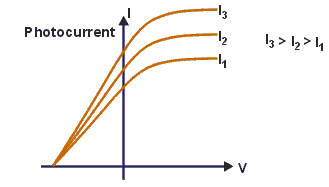(a) Presence of current for zero value potential indicates that the electrons are ejected from the surface of emitter with some energy.

(b) A gradual change in the number of electrons reaching the collector due to change in its potential indicates that the electrons are ejected with a variety of velocities.

(c) Current is reduced to zero for some negative potential of collector indicating that there is some upper limit to the energy of electrons emitted.

(d) Current depends upon the intensity of incident light.

(e) Stopping potential is independent of the intensity of light.

Effect of intensity of light:- The photoelectric currentis directly proportional to theintensity of incident radiation.

Effect of frequency of light:-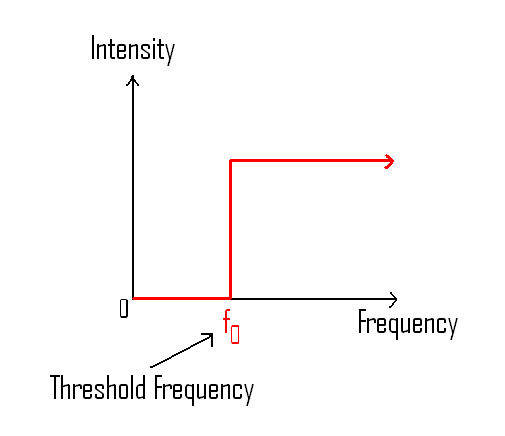?(a) Stopping potentialdepends upon thefrequency of light. Greater the frequency of light greater is the stopping potential.

(b) Saturation current is independent of frequency.

(c) Threshold frequency is the minimum frequency, that capable of producing photoelectric effect.

Laws of Photoelectricity:-(a) Photoelectric effect is an instantaneous process.

(b) Photoelectric current is directly proportional to the intensity of incident light and is independent of its frequency.

(c) The stopping potential and hence the maximum velocity of the electrons depends upon the frequency of incident light and is independent of its frequency.

(d) The emission of electrons stops below a certain minimum frequency known as threshold frequency.

Energy contained in bundle or packet:-

E = hf = hc/λ

Here h is the Planck’s constant and f is the frequency.

Work function:- It is defined as the minimum energy required to pull an electron out from the surface of metal. It is denoted by W0.

Einstein’s equation of photoelectric effect:-

(a) ½ mvmax2 = hf – W0

(b) ½ mvmax2 = hf – hf0 = h(f- f0) = h [c/λ – c/λ0]

(c) eV0 = hf - W0

(d)V0 = [(h/e)f] – [W0/e]

Here f0 is threshold frequency.

Threshold frequency (f0):- f0 = work function/h = W/h

Maximum kinetic energy of emitted photo electrons:-

?Kmax= ½ mvmax2 = eV0

Threshold wavelength:- λ0 = c/f0 = hc/hf0 = hc/W

Slope of V0~ v graph:- Slope= h/e

Rest mass of photon = 0, Charge = 0

Energy of photon:- E = hf = hc/λ

Momentum of photon:- p = E/c = h/λ = hf/c

Mass od photon:- m = E/c2 = h/cλ = hf/c2

For electron, λe = [12.27/√V]Å

For proton, λp = [0.286/√V]Å

For alpha particle, λα = [0.286/√V]Å

For particle at temperature T, λ = h/√3mKT   (E = 3/2 KT)

The wavelength of electron accelerated by potential difference of V volts is:-

λe= [12.27/√V]Å

Number of photons:-

(a) Number of photons per sec per m2, np = Intensity/hf

(b) Number of photons incident per second, np = Power/hf

(c) Number of electrons emitted per second = (efficiency per surface)× (number of photons incident per second)

Compton wave length:-

(a) λc = h/m0c

Here h is the Planck’s constant, m0 is the rest mass of electron and c is the speed of light.

(b) Change in wavelength:- λ' – λ =λc (1-cos?)

de Broglie wavelength (λ):- λ = h/mv = h/√(2mE) = h/√(2meV)

In accordance to Bohr’s postulate of atomic structure, the angular momentum of an electron is an integral multiple of h/2π.

So, mvr = nh/2πBragg’s diffraction law:- 2dsinθ = nλ

Here λ is the wavelength of electron and d is distance between the planes.

Rutherford’s atomic model (α-particle scattering):-

(a) N(θ) ∝ cosec4(θ/2)

(b) Impact parameter, b = [(Ze2) (cot θ/2)]/[(4πε0)E]

Here, E = ½ mv2 = KE of theα particle.

(c) Distance of closest approach, r0 = 2Ze2/(4πε0)E

Here E = ½ mv2 = KE of the α particle.

Bohr’s atomic model:-

(a) The central part of the atom called nucleus, contains whole of positive charge and almost whole of the mass of atom. Electrons revolve round the nucleus in fixed circular orbits.

(b) Electrons are capable of revolving only in certain fixed orbits, called stationary orbits or permitted orbits. In such orbits they do not radiate any energy.

(c) While revolving permitted orbit an electron possesses angular momentum L (= mvr) which is an integral multiple of h/2π.

L=mvr =n (h/2π)

Here n is an integer and h is the Planck’s constant.

(d) Electrons are capable of changing the orbits. On absorbing energy they move to a higher orbit while emission of energy takes place   when electrons move to a lower orbit. If f is the frequency of radiant energy,

hf= W2-W1

Here W2 is the energy of electron in lower orbit and W1 is the energy of electron in higher orbit.

(e) All the laws of mechanics can be applied to electron revolving in a stable orbit while they are not applicable to an electron in  transition.

Bohr’s Theory of Atom:-

(a) Orbital velocity of electron:- vn= 2πkZe2/nh

For a particular orbit (n= constant), orbital velocity of electron varies directly as the atomic number of the substance.

vn∝Z

(b) For a particular element (Z= constant), orbital velocity of the electron varies inversely as the order of the orbit.

vn∝1/n

(c) v = nh/2πmr

Relation between vnand v1:-vn = v1/n

?r= n2h2/4π2kmZe2

So, r∝n2

For, C.G.S system (k = 1), r = n2h2/4π2mZe2

S.I (k = 1/4πε0), r =(ε0/π) (n2h2/mZe2)

Kinetic energy of the electron:- It is the energy possessed by the electron by virtue of its motion in the orbit.

K.E = ½ mv2 = ½ k (Ze2/r)

Potential energy:- It is the energypossessed by the electronby virtue of its position near the nucleus.

P.E = -k (Ze2/r )

Total energy:-

W= K.E + P.E

W=- ½ k (Ze2/r) = -k2 2π2Z2me4/n2h2

For, C.G.S (k = 1), W = - [2π2Z2me4/n2h2]

For, S.I. ( k = 1/4πε0), W = - (1/8ε02) [Z2me4/n2h2]

Since, W∝1/n2, a higher orbit electron possesses a lesser negative energy (greater energy) than that of a lower orbit electron.

Frequency, wavelength and wave number of radiation:-

Frequency, f = k2[2π2Z2me4/h3] [1/n12 – 1/n22]Here R is the Rydberg’s constant and its value is,

R= k2 [2π2Z2me4/ch3]

Bohr’s theory of hydrogen atom (Z=1):-

r= n2h4/4π2me2 (C.G.S)

r= (ε0/π) (n2h2/me2) (S.I)

(b) Energy of electron:-

W= 2π2me4/n2h2    (C.G.S)

W =(1/8ε0)[me4/n2h2]

(c) Frequency, wavelength and wave number of radiation:-

C.G.S:- k =1 and Z=1

Frequency= f=2π2me4/h3 [1/n12 – 1/n22]

Wave number = 1/λ = 2π2me4/ch3 [1/n12 – 1/n22]

S.I:- k =1/4πε0 and Z=1

Frequency= f = (1/8ε0) (me4/h3)[1/n12 – 1/n22]

Wave number = 1/λ = (1/8ε02) (me4/ch3)[1/n12 – 1/n22]

Rydberg’s constant:-

R=k2 =2π2z2 me4/ch3

For hydrogen atom, Z = 1, R = RH = k2 (2π2 me4/ch3).

For C.G.S system (k=1), RH = 2π2 me4/ch3

For S.I system (k=1/4πε0), RH = (1/8ε02) (me4/ch3)

Wave number, 1/λ = RH [1/n12 – 1/n22]

Hydrogen Spectrum:-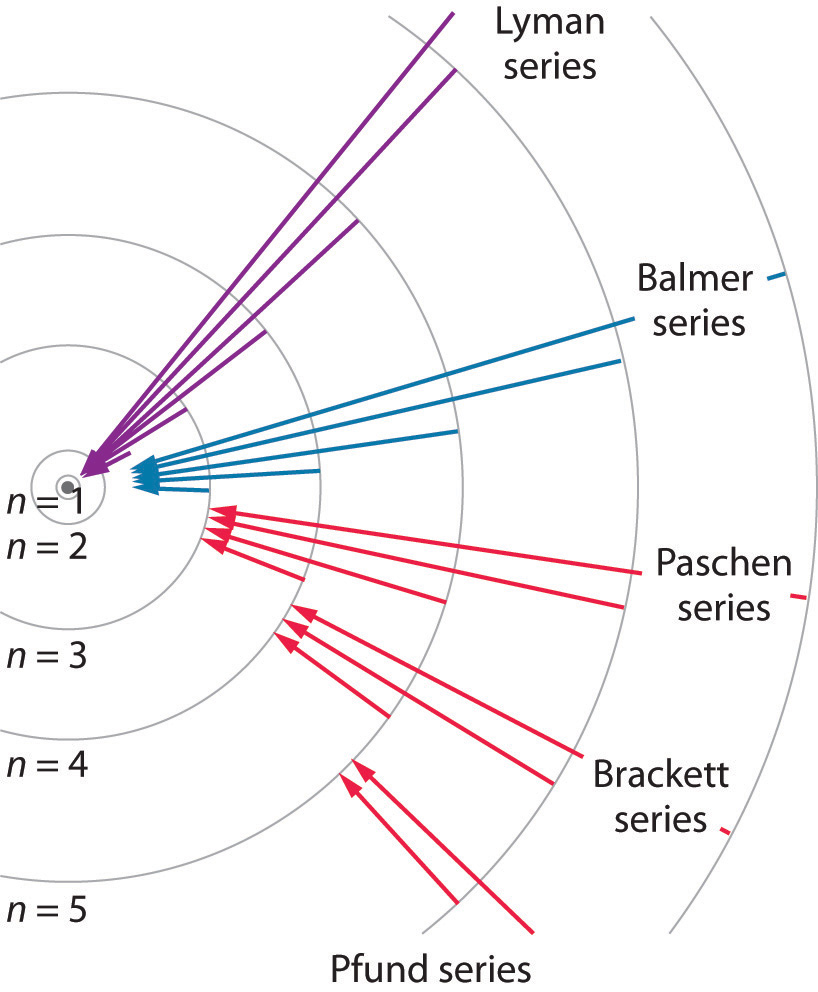(a) For Lyman series:- 1/λ = R [1– 1/n2], n = 2,3,4…..∞

(b) For Balmer series:- 1/λ = R [1/22 – 1/n2], n =3,4,5…..∞

(c) For Paschen series:-1/λ = R [1/32 – 1/n2], n =4,5,6…..∞

(d) For Brackett series:-1/λ = R [1/42 – 1/n2], n =5,6,7…..∞

(e) P-fund series:-1/λ = R [1/52 – 1/n2], n =6,7,8…..∞

Series limits (λmin):-

(a) Lyman:- λmin = 912 Å

(b) Balmer:-λmin = 3645 Å

(c) Paschen:- λmin = 8201 Å

Energy levels of hydrogen atom:-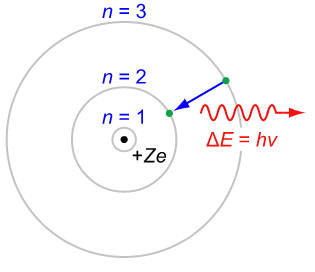W = -k22π2me4/n2h2

For, n=1, W1 = -13.6 eV

For the first excited state, n=2, W2 =W1/4 = (-13.6/4) eV = -3.4 eV

For the second excited state, n=3, W3 =W1/9 = (-13.6/9) eV = -1.51 eV

Similarly, for other excited states, W4 = -0.85 eV and W5 = -0.54 eV

Number of emission lines from excited state:-n = n(n-1)/2

Ionization energy:-

- E1 = +(13.6Z2)eV

(a) For H-atom, I.E = 13.6 eV

(b) For He+ ion, I.E = 54.4 eV

(c) For Li++ ion, I.E = 122.4 eV

Ionization potential:-

(a) For H-atom, I.P = 13.6 eV

(b) For He+ ion, I.P = 54.42 eV

Nuclear Physics

Nuclear Physics:- Branch of physics dealing with the study of nucleus is called nuclear Physics.

Constituents of nucleus (Nucleons) :-

(a) Protons:-

Mass of proton, mp = 1.6726×10-27 kg

Charge of proton = 1.602×10-19 C

(b) Neutron:-

Mass of neutron, mn = 1.6749×10-27 kg

(c) 1 atomic mass unit (1 amu) = 1.66×10-27 kg

(d) 1 amu = 1 u = 931.5 MeV

Properties of nucleus:-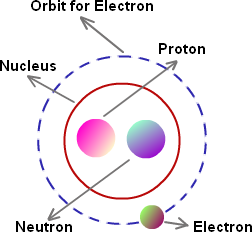(a) Charge on nucleus = 1.602×10-19 Z coulomb

(b) Size of nucleus:-

The radius r of the nucleus depends upon the atomic mass A of the element.

r =R0A1/3

Here R0 = 1.2×10-13 cm     (1 Fermi =10-13 cm)

Volume of the nucleus:-

V = 4/3 πr3 = 4/3 π (R0A1/3)3

(c) Density of nucleus:-

Density of nucleus = mass/volume = A/(4/3 πR03A) = [3/(4πR03)]

Isotopes:- Nuclei having same atomic number Z but different mass number A are called isotopes.

Isobars:- Nuclei having same mass number A but different atomic number Z are called isobars.

Isotones:- Nuclei having the same number of neutrons (N) but different atomic number (Z) are called isotones.

Nature of nuclear force:-

(a) Nuclear forces are attractive in nature.

(b) Nuclear forces are charge independent.

(c) These are short range forces.

(d) Nuclear forces decrease very quickly with distance between two nucleons.

(e) Nuclear forces are spin dependent.

Mas defect:- If the mass of the nucleus HZA is M, then the mass defect,

ΔM = [Zmp + (A-Z)mn – M]?

Here, mp and mn are the masses of the proton and neutron respectively.

Binding Energy:-

Binding Energy = (ΔM) c2 = [Zmp + (A-Z)mn – M] c2

If we use atomic mass instead of nuclear masses, then,

Binding Energy, B = [ZmH + (A-Z)mn – Mat] c2

Here Mat is the mass of the atom  and mH is the mass of the hydrogen atom.

Binding energy per nucleon:-

B/A = [Zmp + (A-Z)mn – M] c2 /A

If we use atomic mass instead of nuclear masses, then,

B/A = [ZmH + (A-Z)mn – Mat] c2/A

Radioactivity:- The phenomenon by virtue of which substance, spontaneously, disintegrate by emitting certain radiations is called radioactivity.

?Alpha decay:-

?It is the process in which a parent nucleus decays into the daughter nucleus by ejecting an alpha particle.

?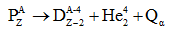Beta decay:- It is the process in which a parent nucleus decays into the daughter nucleus by ejecting an electron.

?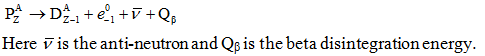Gama decay:- Sometimes the daughter nucleus is left in the excited state. It decays in to any other lower state or ground state by emitting γ-rays.

?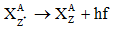(a) Radioactivity is due to the disintegration of a nucleus.

(b) Rate of disintegration is not affected by the external conditions like temperature and pressure etc.

(c) Law of conservation of charge holds good in radioactivity.

(d) The disintegration is accompanied by the emission of energy in terms of α, β and γ-rays either single or all at a time.

Emission of α- particle results in a decrease in its atomic number by 2 and a decrease in its atomic weight by 4.

Emission of β- particle results in an increase in its atomic number by 1 while its atomic weight remains unaffected.

Emission of γ- rays results neither in a change of atomic number nor in a change of atomic weight.

(e) Each of the product disintegration is a new element having physical and chemical properties different from those of the parent atom.

(f) Rate of disintegration of the radioactive substance, at any instant, is directly proportional to the number of atoms present at that instant.

N = N0e-λt

If, t = 1/λ, then, N = N0/e

Therefore, radioactive decay constant is defined as the reciprocal of time in which the number of atoms of radioactive sample is reduced to N0/e.

Half-life (T1/2):- Half-life of a radioactive substance is defined as the time during which the number of atoms of the substance are reduced to half their original value.

T1/2 = 0.693/λ

Thus, half-life of a radioactive substance is inversely proportional to its radioactive decay constant.

Average life (Tav):- Arithmetic mean of the lives of all the atoms is known as mean life or average life of the radioactive substance.

Tav = sum of lives of all atoms / total number of atoms

Average life of a radioactive substance is equal to the reciprocal of its radioactive decay constant.

Relation between T1/2 and Tav:-

T1/2 = 0.693× Tav

Half-life = 0.693×average life

(a) Curie (Ci):- Radioactivity of a substance is said to be one curie if its atoms disintegrate at the rate of 3.7×1010 disintegrations per second.

(b) Rutherford (rd):- Radioactivity of a substance is said to be 1 Rutherford if its atoms disintegrate at the rate of 106 disintegrations per second.

Relation between Curie and Rutherford:-

1 C = 3.7×104 rd

Nuclear fission:-

?Nuclear fission is the process by  which a nucleus breaks up in such a way that the two products obtained are of comparable sizes.

Fission of U92235 by fast moving neutrons is represented as,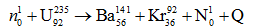Value of Q in this reaction is 200.4443 MeV.

Chain reaction:-The above quoted fission reaction proceeding in an uncontrolled manner is known as chain reaction and forms the basis of atom bomb. Following conditions must be met with, for the chain reaction to proceed.

(a) The fissionable material should be larger than a certain minimum size.

(b) The material should be highly pure.

Nuclear reaction:- Fission reaction of  U92235can be made to proceed in a controlled manner by slowing down the three emitted neutrons. This is achieved by passing them through moderators. Heavy water and carbon rods can be used as moderators. A device in which the fission proceeds in a controlled manner is called a nuclear reaction.

Nuclear fusion:-

?A fusion reaction is one in which two lighter nuclei get fused together to form a heavy nucleus. It is a highly exothermic reaction and produces energy, on a scale, much higher than that produced in fission.

?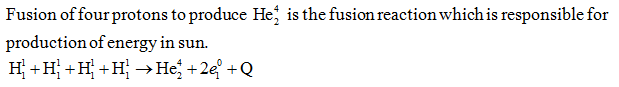Decay constant for two channels:-

(a) λ= λ1+ λ2

(b) T = T1T2/T1+T2

Gamma intensity absorption:-

I = I0e - μx

Laws governing nuclear reaction:-

(a) Law of conservation of charge

(b) Law of conservation of number of nucleons

(c) Law of conservation of energy.

Classification of nuclear reaction:- Nuclear reaction can be classified into following categories.

(a) Elastic scattering

(b) Inelastic scattering

(c) Simple capture

(d) Disintegration

Q-value of nuclear reaction:-

Q= {(mi+mt) – (mp+m0)} c2

Radioisotope:- An isotope, obtained in process of artificial transmutation, which exhibits radioactivity is called a radio-isotope. It can be put to following uses:

(a) Tracer

(b) In medicine

(c) In industry
```### Course Features

• 728 Video Lectures
• Revision Notes
• Previous Year Papers
• Mind Map
• Study Planner
• NCERT Solutions
• Discussion Forum
• Test paper with Video Solution